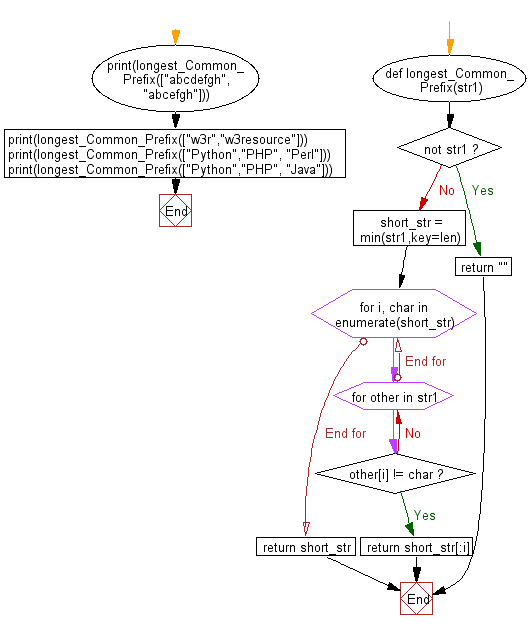﻿ Python: Find the longest common prefix string amongst a given array of strings - w3resource

# Python: Find the longest common prefix string amongst a given array of strings

## Python Basic - 1: Exercise-70 with Solution

Write a Python program to find the longest common prefix string among a given array of strings. Return false if there is no common prefix.
For Example, longest common prefix of "abcdefgh" and "abcefgh" is "abc".

Sample Solution:

Python Code:

``````def longest_Common_Prefix(str1):

if not str1:
return ""

short_str = min(str1,key=len)

for i, char in enumerate(short_str):
for other in str1:
if other[i] != char:
return short_str[:i]

return short_str

print(longest_Common_Prefix(["abcdefgh","abcefgh"]))
print(longest_Common_Prefix(["w3r","w3resource"]))
print(longest_Common_Prefix(["Python","PHP", "Perl"]))
print(longest_Common_Prefix(["Python","PHP", "Java"]))
``````

Sample Output:

```abc
w3r
P
```

Pictorial Presentation:Flowchart:Python Code Editor:

Have another way to solve this solution? Contribute your code (and comments) through Disqus.

What is the difficulty level of this exercise?

Test your Programming skills with w3resource's quiz.

﻿